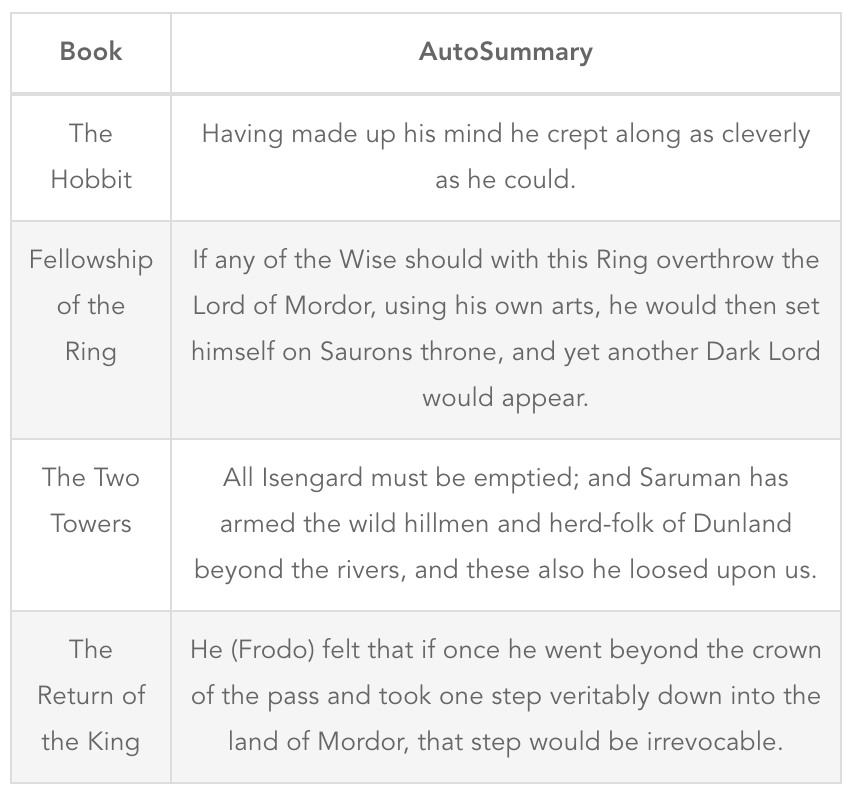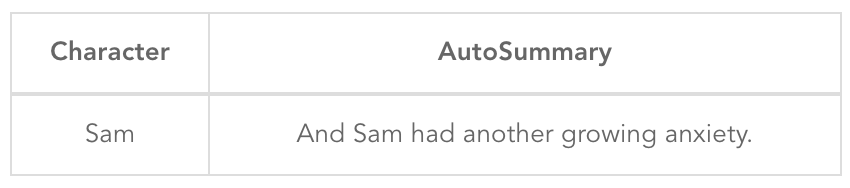# RandomlyUnique

## A Token of Tolkien

The Hobbit and The Lord of the Rings are undeniably great books, with rich complex characters and a deep thoughtful story. So, this seems like an ideal case to apply an autosummary algorithm!

The method I programmed is pretty straightforward, where I find the most representative sentence within a given blob of text. This is accomplished by tokenizing each sentence, converting to a tf-idf matrix, multiplying this matrix by it's transpose to get a similarity between sentences, mapping to a graph where each node is a sentence and each edge is its similarity, then PageRanking to determine the 'best' sentence.

```from nltk import sent_tokenize
from nltk.corpus import stopwords
from operator import itemgetter
from networkx import from_numpy_matrix, pagerank
from sklearn.feature_extraction.text import CountVectorizer, TfidfTransformer

MIN_SENT_LENGTH = 3
FILE_PATH = 'PATH_TO_LORD_OF_THE_RINGS_TEXT'

STOPWORDS = stopwords.words("english")

'''
Opens up the Lord of the Rings file and cleans up the text
'''
encoded_document = document.decode('unicode_escape').encode('ascii', 'ignore')
return encoded_document.lower()

def setup_sentences(document):
'''
Tokenizes the document into sentences, then filters for short sentences
'''
sentences = sent_tokenize(document)
sentences = filter(lambda sent: len(sent.split()) > MIN_SENT_LENGTH, sentences)

return sentences

def rank_sentences(sentences):
'''
Ranks sentences using a page rank algorithm
'''
word_counts = CountVectorizer(
min_df=1E-3,
stop_words=STOPWORDS,
analyzer='word',
).fit_transform(set(sentences))

tfidf = TfidfTransformer().fit_transform(word_counts)
pairwise_similarity = tfidf * tfidf.T
graph = from_numpy_matrix(pairwise_similarity.toarray())

ranked_sentences = pagerank(graph)
ranked_sorted = sorted(ranked_sentences.items(), key=itemgetter(1), reverse=True)
top_sentences = [sentences[index] for index, score in ranked_sorted[:10]]

def print_results(sentences):
for index, sentence in enumerate(sentences, start=1):
print "\n{0}: {1}".format(index, sentence.capitalize())

if __name__ == "__main__":